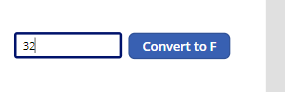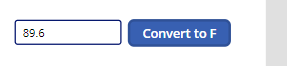# Formula - How to add a button that converts degrees Centigrade to Fahrenheit and vice versa

Here's a walkthrough on how we can customise a data entry screen to include a button that carries out unit measurement conversions..

A common scenario we might encounter is to provide some method for users to convert units of measurement.

This post describes how we can build a screen where a user can enter a value through a text input control. On clicking a button, the app carries out a conversion and replaces the value in the text input control with the converted value.

This type of feature is ideally suited for data entry screens where users may need to perform conversions prior to input.

## Explanation of Maths

The maths to carry out the conversion is shown below. This is the logic that we'll be using in our app.

`//To convert degrees celsius to fahrenheit:F = C * 9/5 + 32//To convert degrees fahrenheit to centigrade:C = (F-32) * 5/9`

## Building the screen

Here are the steps to build the data entry elements on the screen.

First, we add a text input control (txtInput) and a button on our screen.

We set the Default property of txtInput to the following variable:
`locResult`
To configure the button to convert from degrees Celsius to Fahrenheit, we would set the OnSelect property to the following:
`UpdateContext({locResult:(Value(txtInput.Text) * (9/5)) + 32 })`
This is how the design time view of the app appears:If instead, we want to convert from Fahrenheit to Celsius, we would set the OnSelect property to the following:
`UpdateContext({locResult:(Value(txtInput.Text) -32)* (5/9) })`

## Testing the app

At this stage, we can run and test the app. The user can enter a centigrade value like so.On clicking the button, the app converts and overwrites the input value with the Fahrenheit value.## Conclusion

In this post, we walked through how to build a feature on a data entry screen to enable users to easily convert units of measurement.